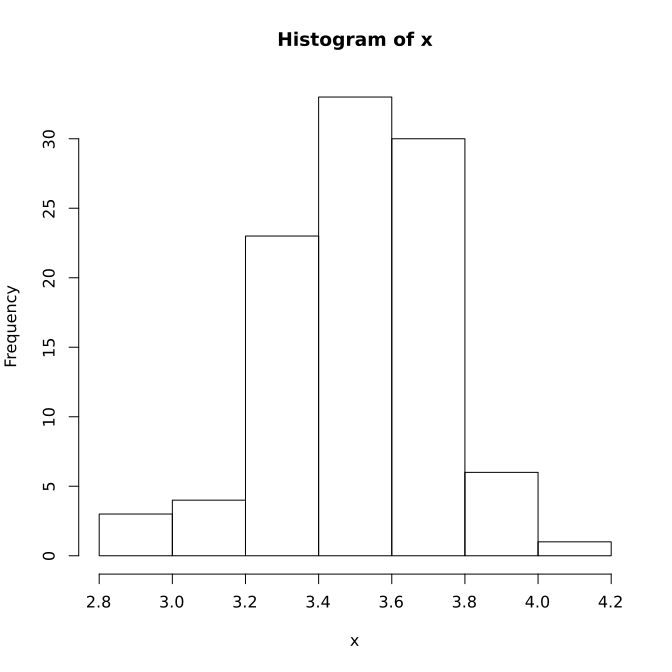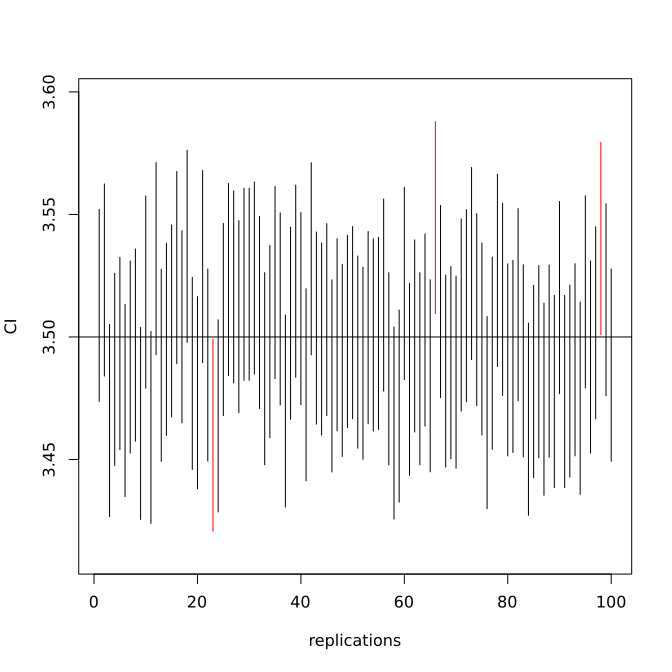# Confidence Interval

Psychological Science is a prestigious journal for psychological research. Its submission guidelines consist of specific guideline on the use of NHST:

Effective January 2014, Psychological Science recommends the use of the "new statistics" - effect sizes, confidence intervals, and meta-analysis - to avoid problems associated with null-hypothesis significance testing (NHST).

Confidence interval provides an alternative method to NHST, which some have argued provides more information on the NHST. A confidence interval (CI) is a type of interval estimate, instead of a point estimate, of a population parameter.

## Formal definition

Let $\theta$ denote a population parameter (unknowns) and $X$ denote a random variable (e.g., GPA) from which the data can be observed. Assume the observed outcome for $X$ is $x$. We can calculate an interval $[l(x),u(x)]$ based on the observed data. More generally, we can define

$\Pr(l(X) \leq \theta \leq u(X))=1-\alpha=C$.

Then $[l(X),u(X)]$ is a confidence interval with confidence level $1-\alpha=C$, or $100(1-\alpha)\%$. $l(X)$ and $u(X)$ are called confidence limits (bounds), lower limit and upper limit, respectively.

### Remarks

• A CI is an observed interval calculated based on a set of observed data. In general, it is different from sample to sample. Therefore, for two studies on the same topic, the CIs can be very different even following exactly the same study design.
• Different from the point estimate, a CI consists of a range of potential values as good estimates of the unknown population parameter.
• For a given CI, it either includes or does not include the population parameter value. Therefore, a CI does not necessarily cover the true parameter values at all.
• If we conduct many separate data analyses of repeated experiment and each time we calculate a CI, the proportion of such intervals that contain the true value of the parameter matches the confidence level C ($1-\alpha$), This is called confidence level.
• When we say, "we are 99% confident that the true value of the parameter is in our confidence interval", we express that 99% of the observed confidence intervals will contain the true value of the parameter.
• The desired level of confidence is set by the researchers, not determined by data. If a corresponding hypothesis test is performed, the confidence level is the complement of respective level of significance, i.e. a 95% confidence interval reflects a significance level of 0.05.

## How to obtain a confidence interval

The basic idea to get a CI is straightforward in theory but can be very difficult in practice. It involves three steps:

1. Obtain a point estimate $\hat{\theta}$ for $\theta$. Note that $\hat{\theta}$ is a function of $x$ or your data.
2. Find out the sampling distribution of $\hat{\theta}$.
3. An equal-tail confidence interval with 95% confidence level can be constructed using the 2.5th and 97.5th percentiles of the sampling distribution.

### An example

Suppose we want to estimate and obtain the confidence interval estimate of the average GPA ($\mu$) of all undergraduate students at University of Notre Dame. GPA typically follows a normal distribution $X\sim N(\mu,\sigma)$. Instead of going out to collect data from students, we will simulate (generate) some data for our example. To simulate data, we need to know the population mean and standard deviation of GPA. Here we assume the mean is $\mu=3.5$ and the standard deviation $\sigma=.2$. Furthermore, we would like to generate a sample of data with the sample size 100.

In R, to generate random number from a normal distribution, the function rnorm() can be used. Specifically for this example, the code x<-rnorm(100,3.5,0.2) generates 100 values from a normal distribution with mean 3.5 and standard deviation 0.2. Therefore, in the function, the first number is the number of the values to generate, the second is the mean and the third is the standard deviation. The code below generates the values, prints them in the output, and displays the histogram of the generated data. Note that the histogram shows a bell shape.

> x<-rnorm(100,3.5,0.2)
> x ## show x
 3.334000 3.586837 3.359713 3.524029 3.447670 3.368659 3.375683 3.549234
 3.654781 3.667078 3.382636 3.231061 3.265321 3.543612 3.508240 3.719976
 3.810934 3.401276 3.540178 3.435721 3.836820 3.527963 3.367449 3.282790
 3.684809 3.746624 3.676275 3.691510 3.359611 3.174088 3.503263 3.724812
 3.709836 4.136255 3.554183 3.435994 3.512146 3.391283 3.320681 3.693763
 3.363223 3.816180 3.536341 3.287929 3.468621 3.684756 3.681145 3.409627
 3.695873 3.313115 3.409239 3.306808 3.765370 3.280114 3.655706 3.718136
 3.706299 3.558405 3.718321 3.880794 3.568745 3.520628 3.653579 3.055296
 3.217441 3.271952 3.799409 3.400029 3.600566 3.234875 3.749574 3.624902
 3.422975 3.673681 3.451874 3.809673 3.442798 3.434386 3.699813 3.486470
 3.187778 3.432287 3.253338 3.600950 2.868837 2.980158 3.548014 3.453090
 2.961468 3.741704 3.530058 3.793508 3.540110 3.834930 3.107434 3.745801
 3.363361 3.483301 3.348338 3.601043
> hist(x) ## histogram
>With the simulated data for 100 students, an estimate of the average GPA ($\theta$) is $\bar{x}=\frac{1}{100}\sum_{i=1}^{100}x_{i}.$ Based on the central limit theorem, if the population variance $\sigma$ is known, regardless of the shape of the population distribution, $\bar{x}$ is at least approximately normally distributed with mean ($\mu$) and standard deviation (standard error of the mean) $s.e.(\bar{x})=\sqrt{\frac{1}{n}\sigma^{2}}=.1\sigma.$ Now we the point estimate is $\hat{\theta}=\bar{x}$ and its sampling distribution is a normal distribution. Then a 95% equal-tail confidence interval can be constructed using the 2.5% and 97.5% percentile of the normal distribution as $[\Phi^{-1}(0.025), \Phi^{-1}(0.975)]$ where $\Phi$ is the normal distribution function. The whole procedure to calculate a CI for a set of simulated data is shown below.
> x <- rnorm(100,3.5,0.2)
> xbar <- mean(x)
> s.e. <- 0.2/10
> qnorm(c(.025, .975), xbar, s.e.)
 3.468670 3.547069
>


Now, try run the code above one more time. Do you get the same confidence interval?

## An experiment for CI interpretation

A CI changes each time with a study. If we repeat the same study again and again, $100(1-\alpha)\%$ of the time the obtained confidence intervals would cover the true population parameter value. This can be shown through a simulation study or experiment. Using the GPA example, we can conduct an experiment using the following steps:

1. Generate a set of GPA data with 100 students from the population
2. Calculate the observed sample mean of GPA and the standard error of x bar
3. Calculate the confidence interval
4. Check whether the confidence interval covers the population parameter value
5. Repeat (1)-(4) 1000 times and count the total number of times that the confidence intervals cover the population value.
6. For a 95% CI, one would expect about 950 times the CIs cover the population value.

The R code below carries out the experiment. The output shows that the among the 1000 sets of CIs calculated based on the 1000 sets of simulated data, 949 of them cover the population value 3.5.

> count<-0
>
> for (i in 1:1000){
+   x<-rnorm(100, 3.5, .2)
+   xbar<-mean(x)
+   s.e.<-.2/10
+   l<-qnorm(.025, xbar, s.e.)
+   u<-qnorm(.975, xbar, s.e.)
+   if (l<3.5 & u>3.5){
+     count<-count+1
+   }
+ }
> count
 949
>


For a given CI, it either covers the population value or not. This can be best demonstrated by plotting the CIs. The R code and output are given below. In the code, we generate 100 CIs, among which 97 cover the population value and 3 do not.

> count<-0
> all.l<-all.u<-NULL
> for (i in 1:100){
+   x<-rnorm(100, 3.5, .2)
+   xbar<-mean(x)
+   s.e.<-.2/10
+   l<-qnorm(.025, xbar, s.e.)
+   u<-qnorm(.975, xbar, s.e.)
+   if (l<3.5 & u>3.5){
+     count<-count+1
+   }
+   all.l<-c(all.l, l)
+   all.u<-c(all.u, u)
+ }
> count
 97
>
> ## generate a plot
> plot(c(1,1), c(all.l, all.u), type='l',
+      ylim=c(min(all.l)-.01, max(all.u)+.01),
+      xlim=c(1,100), xlab='replications',
+      ylab='CI')
> abline(h=3.5)
> for (i in 2:100){
+   if (all.l[i]<3.5 & all.u[i]>3.5){
+     lines(c(i,i), c(all.l[i], all.u[i]))
+   }else{
+     lines(c(i,i),c(all.l[i], all.u[i]),col='red')
+   }
+ }
>## Confidence interval and hypothesis testing

Confidence intervals do not require a-priori hypotheses, nor do they test trivial hypotheses. A confidence interval provides information on both the effect and its precision. A smaller interval usually suggests the estimate is more precise. For example, [3.3, 3.7] is more precise than [3,4].

A confidence interval can be used for hypothesis testing. For example, suppose the null hypothesis $\theta=\theta_{0}$ for any value of $\theta_{0}$. If a confidence interval with confidence level $C=1-\alpha$ contains $\theta_{0}$, we fail to reject the corresponding null hypothesis at the significance level $\alpha$. Otherwise, we reject the null hypothesis at the significance level $\alpha$.

For example, suppose we are interested in testing whether a training intervention method is effective or not. Based on a pre- and post-test design, we find the confidence interval for the change after training is [0.7, 1.5] with the confidence level 0.95. Since this CI does not include 0, we would reject the null hypothesis that the change is 0 at the alpha level 0.05.

Using CI for hypothesis testing does not provide the exact p-value. However, a CI can be used to test multiple hypotheses. For example, for any null hypothesis that the change score is less than 0.7, one would reject it.

CI kinds of focuses on the alternative hypothesis, the effect of interest. It provides a range of plausible values to estimate the effect of interest.

Reichardt and Gollob (1997) discussed conditions that NHST and CI can be useful. NHST is shown generally to be more informative than confidence intervals when assessing (1) the probability that a parameter equals a pre-specified value; (2) the direction of a parameter relative to a pre-specified value (e.g., 0); and (3) the probability that a parameter lies within a pre-specified range.

On the other hand, confidence intervals are shown generally to be more informative than NHST when assessing the size of a parameter (1) without reference to a pre-specified value or range of values, and (2) with reference to many pre-specified values or ranges of values. Hagen (1997) pointed out: "We cannot escape the logic of NHST [null hypothesis statistical testing] by turning to point estimates and confidence intervals" (p. 22). In addition, Schmidt and Hunter (1997) suggested: "The assumption underlying this objection is that because confidence intervals can be interpreted as significance tests, they must be so interpreted. But this is a false assumption" (p. 50).

To cite the book, use: Zhang, Z. & Wang, L. (2017-2022). Advanced statistics using R. Granger, IN: ISDSA Press. https://doi.org/10.35566/advstats. ISBN: 978-1-946728-01-2.